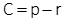## 2. Measuring Parallax

There are two ways to measure parallax depending on object type and accuracy: monoscopic and stereoscopic.

### a. Monoscopic

Parallax direction is parallel with the flight direction. Since the photo x axis is in the direction of flight, parallax can be computed from x coordinates, Figure E-4 and Equation E-1.Figure E-4Parallax Direction

The coordinate system should be based on the flight path since the images shift in that direction (see Chapter D Section 4 of this topic). However unless there is severe course change between photos, using the fiducial system on both photos should be sufficient for most surveying applications.

To visualize parallax distances, Figure E-5 shows the left and right images of points a and b on a single diagram.Figure E-5Parallax determinationEquation E-1

In order to use the monoscopic method, the image point(s) must be distinct on both photographs. Is is extremely difficult, if not impossible, to determine parallax for points in open fields, on water, etc. For those and similar situations, the stereoscopic method must be used.

### b. Stereoscopic

In the stereoscopic method parallax is measured in the 3D view using a floating mark. A floating mark is an artificial object added to both photos so it appears in the 3D view. As the two marks are moved closer together or further apart, it will appear to float higher or lower in the model.
Figure E-6 shows two floating marks on a stereogram.Figure E-6Floating Marks

In the 3D view, they appear at different elevations because of their different parallaxes.

The floating marks are etched on glass plates attached to a measurement scale which allows their spacing to be changed. The device is called a parallax bar, Figure E-7.Figure E-7Parallax Bar

The glass plates are placed on the images, Figure E-8, and their spacing adjusted until the floating mark "sits" on the object in the 3D model. That places the individual marks at correct parallax distance for the elevation.Figure E-8Using a Parallax Bar

The parallax distance is determined using Equation E-2.Equation E-2 C: Parallax bar constantr: scale reading

The constant is determined by using Equation E-1 to determine the parallax of a point, then measuring it with the parallax bar, Equation E-3.Equation E-3

The advantage of a parallax bar it it can be used on indistinct as well as distinct points.